Computational & Technology Resources
an online resource for computational,
engineering & technology publications
Civil-Comp Proceedings
ISSN 1759-3433
CCP: 83
PROCEEDINGS OF THE EIGHTH INTERNATIONAL CONFERENCE ON COMPUTATIONAL STRUCTURES TECHNOLOGY
Edited by: B.H.V. Topping, G. Montero and R. Montenegro
Paper 286

Viscoelastic Analysis of a Bernoulli-Navier Beam Resting on an Elastic Medium

C. Floris and F.P. Lamacchia

Department of Structural Engineering, Politecnico di Milano, Milan, Italy

Full Bibliographic Reference for this paper
C. Floris, F.P. Lamacchia, "Viscoelastic Analysis of a Bernoulli-Navier Beam Resting on an Elastic Medium", in B.H.V. Topping, G. Montero, R. Montenegro, (Editors), "Proceedings of the Eighth International Conference on Computational Structures Technology", Civil-Comp Press, Stirlingshire, UK, Paper 286, 2006. doi:10.4203/ccp.83.286
Keywords: viscoelasticity, Bernoulli Navier beam, elastic media, contact problem, numerical solution, Boussinesq model, Winkler model, analytical solution.

Summary
A Bernoulli Navier beam with viscoelastic behavior is considered. The beam is subject to static loads and rests on an elastic medium. As regards the last, attention is focused on Boussinesq's and Winkler's models. Many engineering materials exhibit a constitutive law depending on time, that is they have a viscous behavior. This can influence the static behaviour of a beam resting on an elastic medium. This is a problem that to the authors' knowledge has had little attention.

As regards the beam, even if it is in a plane stress state and the shear deformability is disregarded, from a theoretical point of view, if the beam is homogeneous, the viscoelastic constitutive law requires the knowledge of two functions, that is the volumetric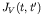and the deviatoric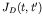creep functions. These functions can be replaced by the uniaxial creep function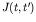and the viscous Poisson ratio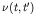. However, for many materials such as concrete the last can be considered independent of time as will be assumed here.

By applying the usual assumptions of linear viscoelasticity and plane section law, the beam equilibrium is governed by the following integrodifferential equation: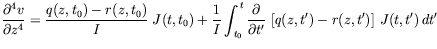(50)

whereis the second moment of the area of the beam transverse section,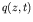the external load, and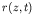the distributed load exchanged by the beam and the elastic medium. By integrating (50) per parts, the alternative form is found: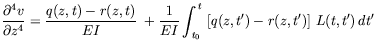(51)

where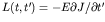is the viscous kernel, and E is the elastic modulus of the beam.

The compatibility between the displacements of the beam axis and the superior surface of the Boussinesq's elastic medium requires that the following integral equation is satisfied: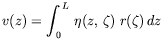(52)

where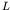is beam length, and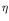the Green function, for which here it assumed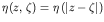. An analytical solution of Equations, (51,52) is hardly conceivable. In the present paper, the problem is discretized in both time and space, and a solution procedure is proposed, which avoids the direct numerical integration of (51). For each time instant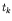a set of algebraic equations is solved of the form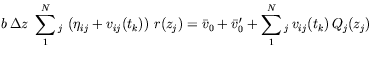(53)

in which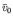,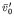are fictitious restraint displacement and rotation,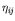,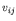are influence coefficients for the soil and the beam, respectively, the last being computed taking creep into account.

A Winkler's medium does not transmit shear, and the interface load is given by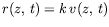(54)

After substituting Equation (54) in (51), separating the variables, a solution is looked for in the form of an infinite series as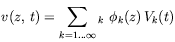(55)

The functions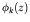must satisfy the boundary conditions, and constitute a complete ortho-normal basis in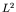. It is emphasized that the eigenfunctions of the free vibrations of the same beam have such features. After some algebra, the infinite set of Volterra's integral equations is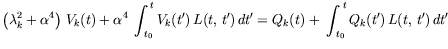(56)

where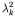are the eigenvalues of the free vibration problem,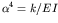,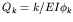and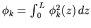. Equation (56) admits an analytical solution. This is given in close form when the creep law is either hereditary or of pure aging type. In this second case, the creep kernel is that suggested by Dischinger, Krall, and Whitney, which depends on the loading time only, that is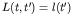. The numerical example compares the responses of a beam resting on a Winkler soil for both hereditary and aging behavior.

purchase the full-text of this paper (price £20)